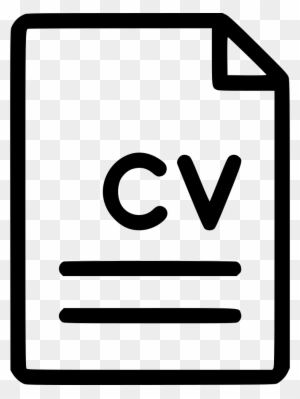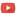KE8QZC | SFW | TSWSyllabus

Homework
For Exam 1
HW1 (due 30 Aug) [soln]: p.8 #1,2,6,7,8,9,16,24,25,30,31,35; p.25 #1,2,3,4,5,6,9,10
(for graduate students): p.8 #18,28,32,33,36; p.25 #11,12
HW2 (due 6 Sep) [soln]: p.8 #24,25,30,31,35; p.25 #1,2,3,4,5,6,9,10,18,19; p.34 #4,5,6,7,11,12
(for graduate students): p.8 #28,32,33,36; p.25 #11,12; p.34 #13,14,15
HW3 (due 13 Sep) [soln]: p.34 #4,5,6,7,11,12,13,14
For Exam 2
HW4 (due 25 Sep): p.36 #26, 27; p.45 #1, 2, 4, 5, 6, 12, 13, 14, 15, 19, 33, 36
(for graduate students): p.36 #28; p.45 #10, 24, 31, 35
HW5 (due 29 Sep): p.55 #4, 5, 6, 7, 8, 9, 11, 12, 23, 24, 27, 28, 41, 43, 51, 54
(for graduate students): #46, 47, 49

Quizzes
For Exam 1
Quiz 1 [soln]: Define a function that is one-to-one, but is not onto.
Quiz 2 (due 8 Sep) [soln]: Complete the following table so that it makes $*$ into a commutative binary operation: $$\begin{array}{l|l|l|l} *&a&b&c \\ \hline a&b&&c \\ \hline b&&b& \\ \hline c&&&b \end{array}$$
Quiz 3 (due 14 Sep) [soln]: Let $*$ be defined on $\mathbb{R}^+=(0,\infty)$ (positive real numbers) by letting $a*b=\sqrt{ab}$. Does the structure $\left\langle \mathbb{R}^+, * \right\rangle$ obey all of the group axioms $\mathscr{G}_1$, $\mathscr{G}_2$, and $\mathscr{G}_3$? If not, then which ones does it not satisfy?
Quiz 4 (due 19 Sep) [soln]: Does the binary operation $*$ defined in the following table obey the group axioms $\mathscr{G}_2$ and $\mathscr{G}_3$ of a group on the set $G=\{a,b,c\}$? Is it a commutative operation? Explain.
$$\begin{array}{l||l|l|l} * &a&b&c \\ \hline\hline a&a&a&b \\ \hline b&b&b&b \\ \hline c&c&b&c \end{array}$$ For Exam 2
Quiz 5 (due 25 Sep) [soln]: Argue why $\pi\mathbb{Z}=\left\{\pi n \colon n \in \mathbb{Z}\right\}$ is a subgroup of $\left\langle \mathbb{R},+\right\rangle$.
For Exam 3

Exams
Exam 1 (20 September)
Exam 2 (18 October)
Exam 3 (15 November)